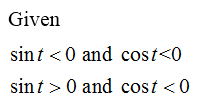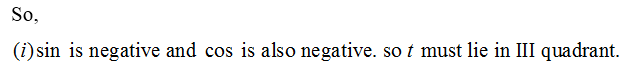# Find the quadrant in which the terminal point detemined by t lies.sin(t)<0 and cos(t)<0sin(t)>0 and cos(t)<0

Question
1 views

Find the quadrant in which the terminal point detemined by t lies.

sin(t)<0 and cos(t)<0

sin(t)>0 and cos(t)<0

check_circle

Step 1Step 2...

### Want to see the full answer?

See Solution

#### Want to see this answer and more?

Solutions are written by subject experts who are available 24/7. Questions are typically answered within 1 hour.*

See Solution
*Response times may vary by subject and question.
Tagged in

### Trigonometry